# Blumberg theorem

(diff) ← Older revision | Latest revision (diff) | Newer revision → (diff)

In 1922, H. Blumberg [a1] proved that if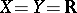, the real number set, then: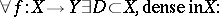(a1)Even for functions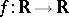, the setin (a1) cannot be made to have cardinality(see [a2]). S. Baldwin (see the references of [a6]) showed that it is consistent with the axioms of set theory that the setin (a1) can always be chosen to be uncountably dense; the setcannot be necessarily chosen so that, for one-to-one functions,is a homeomorphism (C. Goffman; see the references of [a6]); further, the setcannot necessarily be chosen so as to make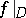differentiable or monotonic (see J. Cedar; see the references of [a6]). Despite the above, J.B. Brown (see the references of [a6]) proved that for everythere exists a set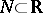,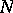being-dense in, such that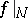is pointwise discontinuous (relative to).

## Blumberg spaces.

Let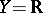. A spaceis called a Blumberg space if (a1) holds for. J.C. Bradford and Goffman [a3] proved that a metric spaceis Blumberg if and only ifis a Baire space, i.e., a space in which open, non-empty subsets are of the second category. The key lemma in their proof is the Banach category theorem. H.E. White [a4] extended the Bradford–Goffman theorem to topological spaceswhich have-disjoint pseudo-bases (cf. also Topological space). He also showed that the real number set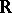with the density topology is a Baire space which is not Blumberg. W.A.R. Weiss (see the references of [a5]) gave an example of a compact Hausdorff space which is not Blumberg. Z. Piotrowski and A. Szymański [a7] showed that ifis a space for which (a1) holds with, then (a1) holds for every second-countable space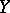. The following characterization is of interest (see [a7]): A space is Blumberg if and only if for every countable covering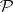ofthere exists a dense subsetofsuch that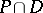is open infor every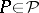, see also [a8] for more characterizations. General references for this area are [a5], [a6].

The simple example of the identity function from the real number set with the Euclidean topology into the real number set with the discrete topology exhibits the necessity of certain restrictions placed upon the range space. Spacesfor which (a1) holds, where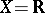, were studied in [a9].

## The dynamics of Blumberg spaces.

It is easy to see that a dense or closed subspace of a Blumberg space need not be Blumberg. The Stone–Čech compactification of a dense subspace of a completely regular Blumberg space is a Blumberg space, a result of R. Levy and R.H. McDowell (see the references of [a7]).

The Cartesian product of Blumberg spaces need not be a Blumberg space, since there is a metric Baire space (hence Blumberg space) whose square is not Baire. On the other hand, S. Todorčević [a10] showed that there is a first-countable compact spacethat is not Blumberg, whereas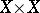is a Blumberg space. It follows from the above theorem that the image of a Blumberg space under an open and continuous function need not be Blumberg.

Consider the unionof the graph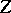of the functiondefined by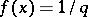if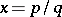in lowest terms,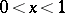, and a copyof the rational number set between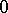and. The natural projection ofonto(which is constant on) shows that even perfect, continuous functions do not necessarily preserve Blumberg spaces. In contrast, Blumberg spaces are preserved in pre-images under irreducible surjections [a7].

M. Valdivia [a11] showed that (a1) holds for linear transformations, whereandare metrizable linear spaces andis of the second category. L. Drewnowski subsequently proved that "dense subset" in Valdivia's theorem cannot be replaced by "dense linear subspace" .

Blumberg sets, i.e. dense setsappearing in (a1), have been studied in connection with characterizations of certain almost-continuous functions, such as quasi-continuous functions ([a13]).

How to Cite This Entry:
Blumberg theorem. Encyclopedia of Mathematics. URL: http://encyclopediaofmath.org/index.php?title=Blumberg_theorem&oldid=18487
This article was adapted from an original article by Z. Piotrowski (originator), which appeared in Encyclopedia of Mathematics - ISBN 1402006098. See original article